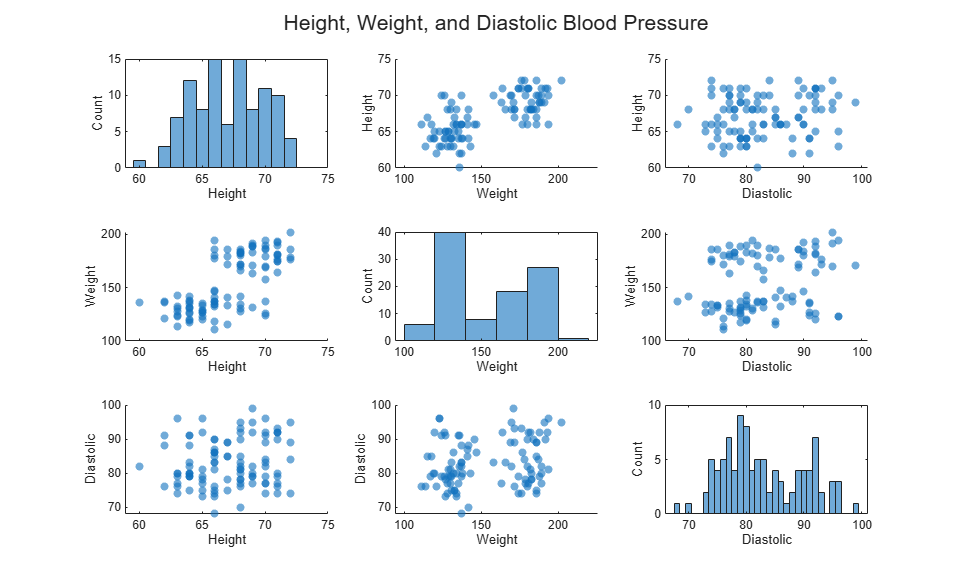# Chart Class for Displaying Variable Size Tiling of Plots

This example shows how to define a class for creating a tiling of plots that can be any size, depending on the size of the user's data. The chart has a public `Data` property that accepts an m-by-n matrix. The chart displays an n-by-n square tiling of scatter plots and histograms. The scatter plots show the different columns of the data plotted against each other. The histograms show the distribution of the values within each column of the data.

The `update` method in this class recreates the histograms and scatter plots to reflect changes in the data. If the grid size of the layout conflicts with the size of the data, then all the axes are deleted and the `GridSize` property is updated to match the size of the data. Then a new set of axes objects is created.

To define the class, copy the following code into the editor and save it with the name `TrellisChart.m` in a writable folder.

```classdef TrellisChart < matlab.graphics.chartcontainer.ChartContainer properties Data(:,:) {mustBeNumeric} ColNames(1,:) string TitleText(1,:) string end methods (Access = protected) function setup(obj) % Use one toolbar for all of the axes axtoolbar(getLayout(obj),'default'); end function update(obj) % Get the layout and store it as tcl tcl = getLayout(obj); numvars = size(obj.Data,2); % Reconfigure layout if needed if numvars ~= tcl.GridSize(1) % Delete layout contents to change the grid size delete(tcl.Children); if numvars>0 tcl.GridSize = [numvars numvars]; for i = 1:numvars^2 nexttile(tcl,i); end end end % Populate the layout with the axes ax = gobjects(numvars,numvars); for col = 1:numvars for row = 1:numvars % Get the axes at the current row/column t = col + (row-1) * numvars; ax(row,col)=nexttile(tcl,t); if col==row % On the diagonal, draw histograms histogram(ax(row,col),obj.Data(:,col)); ylabel(ax(row,col),'Count') else % Off the diagonal, draw scatters scatter(ax(row,col),obj.Data(:,col),... obj.Data(:,row),'filled','MarkerFaceAlpha',0.6) if length(obj.ColNames) >= row ylabel(ax(row,col),obj.ColNames(row)); end end if length(obj.ColNames) >= col xlabel(ax(row,col),obj.ColNames(col)); end end % Link the x-axis for each column, so that panning or zooming % affects all axes in the column. linkaxes(ax(:,col),'x') end % Chart title title(tcl,obj.TitleText,'FontSize',16); end end end ```

After saving the class file, create an instance of the chart.

```load patients chartTitle = "Height, Weight, and Diastolic Blood Pressure"; c = TrellisChart('Data',[Height Weight Diastolic], ... 'colNames', ["Height" "Weight" "Diastolic"],... 'TitleText',chartTitle); ```